Chapter 5. Chemical Composition

# 5.2 The Mole

### Learning Objectives

By the end of this section, you will be able to:

• Define the amount unit mole and Avogadro’s number
• Explain the relation between mass, moles, and numbers of atoms or molecules, and perform calculations to derive these quantities from one another

## Counting by Weighing

Atomic and molecular mass provides a way of understanding/predicting the weights of substances in a reaction. For example, consider the reaction:

C(s)  + O2(g)  →  CO2(g)

This equation tells us that carbon reacts with oxygen gas to produce carbon dioxide in a 1:1:1 ratio.  In other words, the equation tells us that 1 atom of carbon reacts with 1 molecule of Oto form 1 molecule of CO2, or 500 C atoms will react with 500 O2 molecules to form 500 molecules for CO2, and so on. That is, we need an equal number of C atoms and O2 molecules. Now, it’s impractical to actually count out a number of atoms or molecules, they are just too small, but we can do this in effect by weighing.  Looking at an everyday item as an example of counting by weight, see example 1.

### Example 1

How many grams should you weigh to get 5000 nails if each nail has an average mass of 0.25g?

Solution

$5000\;\rule[0.75ex]{2.0em}{0.1ex}\hspace{-2.0em}\text{nails} \times \frac{0.25\;\text{g}}{1\;\rule[0.25ex]{1.25em}{0.1ex}\hspace{-1.25em}\text{nail}} = 1.2\times 10^3\;\text{g are needed}$

Test Yourself

If nails have an average mass of 0.25g, how many nails are present in 62.5g of nails?

2.5×102 nails

Furthermore, if two different types of things are measured by weighing, the same number of each will be present if the ratio of the masses weighed out is equal to the ratio of masses of the individual units.

For example:  If 1 nail weighs 0.25 g and 1 screw weighs 0.50 g, then 300 g of nails will contain the same number of items as 600 g of screws.

Why?  1 screw weighs twice as much as 1 nail, therefore you must have the same number of each item when you weigh out a total mass of screws that’s twice the total mass of nails.

Proof:

$300\;\rule[0.75ex]{0.5em}{0.1ex}\hspace{-0.5em}\text{g nails} \times \frac{1\;\text{nail}}{0.25\;\rule[0.25ex]{0.5em}{0.1ex}\hspace{-0.5em}\text{g nails}} = 1200\;\text{nails}$

$600\;\rule[0.75ex]{0.5em}{0.1ex}\hspace{-0.5em}\text{g screws} \times \frac{1\;\text{screw}}{0.50\;\rule[0.25ex]{0.5em}{0.1ex}\hspace{-0.5em}\text{g screws}} = 1200\;\text{screws}$

It is the same idea, with:

2 kg screws & 1 kg nails, or
2 tons screws & 1 ton nails, or
2 dozen tons of screws & 1 dozen tons of nails…

We know that in every case the number of screws is the same as the number of nails.
Note: in each example, we don’t necessarily know what the number of items is, but we can be sure that it’s the same number of each item.

So, for the above reaction, C(s)  + O2(g)  →  CO2(g), as long as we weigh out amounts of C and Oin the same ratio as the weights of 1 C atom (12.011 amu) and 1 Omolecule (31.9988 amu), we can be sure we’ll have an equal number of C atoms and Omolecules.

Now, the easiest way to choose total weights in the desired ratio—without doing any math—is to just mimic the numerical values of the known atomic and molecular weights. For example, to make sure we have a 12.011 to 31.9988 weight ratio of C and O2, we could just weigh out:

12.011 grams of C and 31.9988 grams of O2
or   12.011 kg of C and 31.9988 kg of O2
or   12.011 lbs of C and 31.9988 lbs of O2
or   12.011 tons of C and 31.9988 tons of O2
or etc..  They all will have equal numbers of C atoms and Omolecules.

## The Mole

The identity of a substance is defined not only by the types of atoms or ions it contains, but by the quantity of each type of atom or ion. For example, water, H2O, and hydrogen peroxide, H2O2, are alike in that their respective molecules are composed of hydrogen and oxygen atoms. However, because a hydrogen peroxide molecule contains two oxygen atoms, as opposed to the water molecule, which has only one, the two substances exhibit very different properties. Today, we possess sophisticated instruments that allow the direct measurement of these defining microscopic traits; however, the same traits were originally derived from the measurement of macroscopic properties (the masses and volumes of bulk quantities of matter) using relatively simple tools (balances and volumetric glassware). This experimental approach required the introduction of a new unit for amount of substances, the mole, which remains indispensable in modern chemical science.

The mole is an amount unit similar to familiar units like pair, dozen, gross, etc. It provides a specific measure of the number of atoms or molecules in a bulk sample of matter.

### Estimating the numerical value of Avogadro’s number

The mole, the SI unit for “substance”, is a unit for counting, and it’s often said that we can think of the mole as like a dozen. A subtle difference, however is that a dozen is defined as a specific number (12) of items, but a mole (Avogadro’s number of items) is defined as whatever number of H atoms you’d have if you weighed out 1.00794 g of H, (or S atoms in 32.066 g of S, or 12C atoms in 12 g of 12C, and so on).

This usually isn’t a problem, as these terms are normally encountered. If we bought a dozen donuts, we’d want to know how many we got—is there one for each person, etc.. But if we weigh out a mole of Na (22.9898 g) and a mole of Cl (35.4527 g), we probably don’t care how many atoms we have—we’re just happy to know we have the same number of atoms of each, in order to make NaCl without any atoms of Na or Cl leftover.

However, in some relatively rare circumstances, we may need to know how many atoms or molecules do we have when we weigh out a certain amount. For this, we’d actually like to know a numerical value for Avogadro’s number. To get this value, someone has to measure how many H atoms are there in 1.00794 g of H, (or S atoms in 32.066 g of S, or 12C atoms in 12 g of 12C, or…). To do that, someone has to measure the mass, in grams, of a single atom.

Many different ways have been dreamed up for estimating, in effect, the mass of a single atom. One very good one is to weigh, in grams, a crystal of a measured volume, and use the diffraction of x-rays to measure the distance between adjacent atoms, and thus estimate the number of atoms in the crystal. Calculating the number of things in a mole (Avogadro’s number) can be illustrated using 12C as an example. 12C provides a good basis because it’s the only substance where we can start with the mass of an atom in amu exactly (12 g).

First, from the definition of a mole:  one 12C  atom weighs 12 amu’s (exactly),
so a mole of 12C means 12 g of 12C (exactly).

The current best measurements give the mass of a 12C atom to 8 significant figures as 1.9926465 x 10-23g, so to determine Avogadro’s number we ask how many times 1.99264654 x 10-23g goes into 12 g (exactly).

This works out as:

$\frac{12\;\rule[0.25ex]{0.5em}{0.1ex}\hspace{-0.5em}\text{g (exactly) /mole}}{1.99264654\times 10^{-23}\;\rule[0.25ex]{0.5em}{0.1ex}\hspace{-0.5em}\text{g/atom}} = 6.0221418\times 10^{23}\;\text{atoms/mole}$

So, based on current best measurements, we can write:

1 Mole of things  = Avogadro’s number of them

Avogadro’s number  =  6.0221418 x 1023  =  6.022 x 1023 approximately

Remember: this value, 6.022… x 1023, refers to entities/particles/atoms/ molecules/ions —whatever you have a mole of—per mole, just like dozen can be for muffins, donuts, cookies, etc….

Therefore, a mole is defined as the amount of substance containing the same number of discrete entities (such as atoms, molecules, and ions) as the number of atoms in a sample of pure 12C weighing exactly 12 g. One Latin connotation for the word “mole” is “large mass” or “bulk,” which is consistent with its use as the name for this unit. The mole provides a link between an easily measured macroscopic property, bulk mass, and an extremely important fundamental property, number of atoms, molecules, and so forth.

The number of entities composing a mole has been experimentally determined to be 6.0221418 × 1023, a fundamental constant named Avogadro’s number (NA) or the Avogadro constant in honor of Italian scientist Amedeo Avogadro. This constant is properly reported with an explicit unit of “per mole,” a conveniently rounded version being 6.022 × 1023/mol.

Consistent with its definition as an amount unit, 1 mole of any element contains the same number of atoms as 1 mole of any other element. The masses of 1 mole of different elements, however, are different, since the masses of the individual atoms are drastically different. The molar mass of an element (or compound) is the mass in grams of 1 mole of that substance, a property expressed in units of grams per mole (g/mol) (see Figure 1).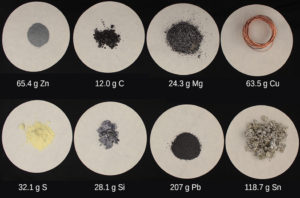Figure 1. Each sample contains 6.022 × 1023 atoms —1.00 mol of atoms. From left to right (top row): 65.4 g zinc, 12.0 g carbon, 24.3 g magnesium, and 63.5 g copper. From left to right (bottom row): 32.1 g sulfur, 28.1 g silicon, 207 g lead, and 118.7 g tin. (credit: modification of work by Mark Ott)

Because the definitions of both the mole and the atomic mass unit are based on the same reference substance, 12C, the molar mass of any substance is numerically equivalent to its atomic or formula weight in amu. Per the amu definition, a single 12C atom weighs 12 amu (its atomic mass is 12 amu). According to the definition of the mole, 12 g of 12C contains 1 mole of 12C atoms (its molar mass is 12 g/mol). This relationship holds for all elements, since their atomic masses are measured relative to that of the amu-reference substance, 12C. Extending this principle, the molar mass of a compound in grams is likewise numerically equivalent to its formula mass in amu (Figure 2).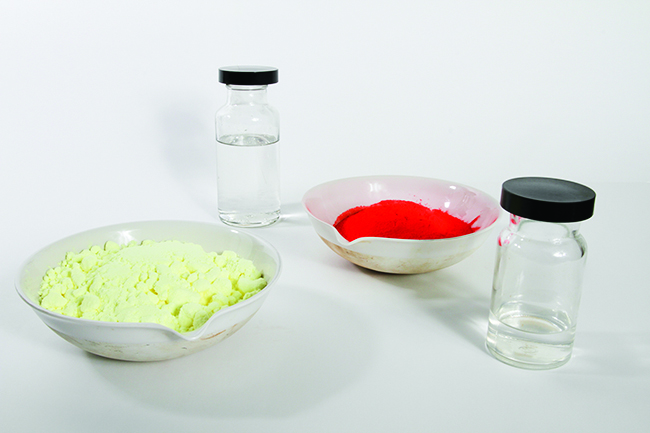Figure 2. Each sample contains 6.022 × 1023 molecules or formula units—1.00 mol of the compound or element. Clock-wise from the upper left: 130.2 g of C8H17OH (1-octanol, formula mass 130.2 amu), 454.4 g of HgI2 (mercury(II) iodide, formula mass 454.4 amu), 32.0 g of CH3OH (methanol, formula mass 32.0 amu) and 256.5 g of S8 (sulfur, formula mass 256.5 amu). (credit: Sahar Atwa)
Element Average Atomic Mass (amu) Molar Mass (g/mol) Atoms/Mole
C 12.011 12.011 6.022 × 1023
H 1.00794 1.00794 6.022 × 1023
O 15.9994 15.9994 6.022 × 1023
Na 22.9898 22.9898 6.022 × 1023
Cl 35.4527 35.4527 6.022 × 1023
Table 1.

While atomic mass and molar mass are numerically equivalent, keep in mind that they are vastly different in terms of scale, as represented by the vast difference in the magnitudes of their respective units (amu versus g). To appreciate the enormity of the mole, consider a small drop of water weighing about 0.03 g (see Figure 3). Although this represents just a tiny fraction of 1 mole of water (~18 g), it contains more water molecules than can be clearly imagined. If the molecules were distributed equally among the roughly seven billion people on earth, each person would receive more than 100 billion molecules.Figure 3. The number of molecules in a single droplet of water is roughly 100 billion times greater than the number of people on earth. (credit: “tanakawho”/Wikimedia commons)The mole is used in chemistry to represent 6.022 × 1023 of something, but it can be difficult to conceptualize such a large number. Watch this video and then complete the “Think” questions that follow. Explore more about the mole by reviewing the information under “Dig Deeper.”

The relationships between formula mass, the mole, and Avogadro’s number can be applied to compute various quantities that describe the composition of substances and compounds. For example, if we know the mass and chemical composition of a substance, we can determine the number of moles and calculate number of atoms or molecules in the sample. Likewise, if we know the number of moles of a substance, we can derive the number of atoms or molecules and calculate the substance’s mass.

### Example 2

According to nutritional guidelines from the US Department of Agriculture, the estimated average requirement for dietary potassium is 4.7 g. What is the estimated average requirement of potassium in moles?

Solution
The mass of K is provided, and the corresponding amount of K in moles is requested. Referring to the periodic table, the atomic mass of K is 39.0983 amu, and so its molar mass is 39.0983 g/mol. The given mass of K (4.7 g) is a bit more than one-tenth the molar mass (39.0983 g), so a reasonable “ballpark” estimate of the number of moles would be slightly greater than 0.1 mol.

The molar amount of a substance may be calculated by dividing its mass (g) by its molar mass (g/mol):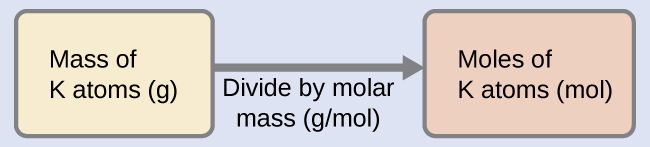The factor-label method supports this mathematical approach since the unit “g” cancels and the answer has units of “mol:”

$4.7 \rule[0.5ex]{0.5em}{0.1ex}\hspace{-0.5em}\text{g} \;\text{K} \times \frac{\text{mol K}}{39.0983 \rule[0.25ex]{0.5em}{0.1ex}\hspace{-0.5em}\text{g}} = 0.12 \;\text{mol of potassium}$

The calculated magnitude (0.12 mol K) is consistent with our ballpark expectation, since it is a bit greater than 0.1 mol.

Test Yourself
Beryllium is a light metal used to fabricate transparent X-ray windows for medical imaging instruments. How many moles of Be are in a thin-foil window weighing 3.24 g?

0.360mol

### Example 3

A liter of air contains 9.2 × 10−4 mol argon. What is the mass of Ar in a liter of air?

Solution
The molar amount of Ar is provided and must be used to derive the corresponding mass in grams. Since the amount of Ar is less than 1 mole, the mass will be less than the mass of 1 mole of Ar, approximately 40 g. The molar amount in question is approximately one-one thousandth (~10−3) of a mole, and so the corresponding mass should be roughly one-one thousandth of the molar mass (~0.04 g):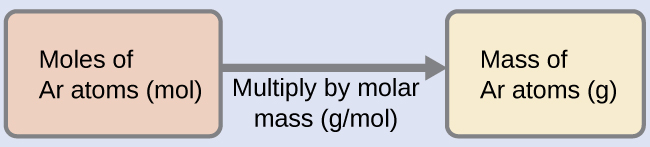In this case, logic dictates (and the factor-label method supports) multiplying the provided amount (mol) by the molar mass (g/mol):

$9.2 \times 10^{-4} \;\rule[0.5ex]{1.75em}{0.1ex}\hspace{-1.75em}\text{mol} \;\text{Ar} \times \frac{39.948 \;\text{g}}{\rule[0.25ex]{1.25em}{0.1ex}\hspace{-1.25em}\text{mol} \;\text{Ar}} = 0.037 \;\text{g of argon}$

The result is in agreement with our expectations, around 0.04 g of argon.

Test Yourself
What is the mass of 2.561 mol of gold?

504.4 g

### Example 4

Copper is commonly used to fabricate electrical wire (Figure 4). How many copper atoms are in 5.00 g of copper wire?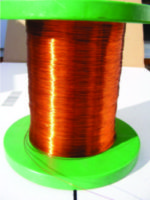Figure 4. Copper wire is composed of many, many atoms of Cu. (credit: Emilian Robert Vicol)

Solution
The number of Cu atoms in the wire may be conveniently derived from its mass by a two-step computation: first calculating the molar amount of Cu, and then using Avogadro’s number (NA) to convert this molar amount to number of Cu atoms: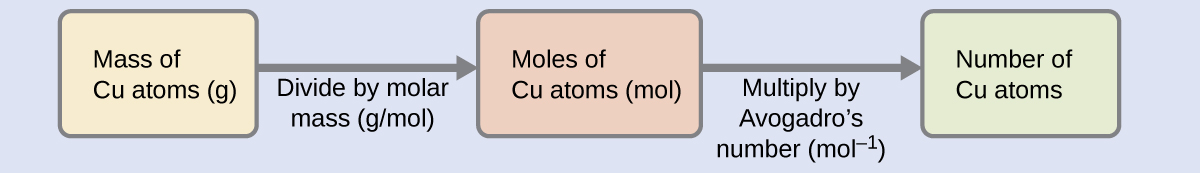Considering that the provided sample mass (5.00 g) is a little less than one-tenth the mass of 1 mole of Cu (~64 g), a reasonable estimate for the number of atoms in the sample would be on the order of one-tenth NA, or approximately 1022 Cu atoms. Carrying out the two-step computation yields:

$5.00 \;\rule[0.5ex]{0.5em}{0.1ex}\hspace{-0.5em}\text{g} \;\text{Cu} \times \frac{\rule[0.25ex]{1.25em}{0.1ex}\hspace{-1.25em}\text{mol} \;\text{Cu}}{63.546 \rule[0.25ex]{0.5em}{0.1ex}\hspace{-0.5em}\text{g}} \times \frac{6.022 \times 10^{23} \;\text{atoms}}{\rule[0.25ex]{1.25em}{0.1ex}\hspace{-1.25em}\text{mol}} = 4.74 \times 10^{22} \;\text{atoms of copper}$

The factor-label method yields the desired cancellation of units, and the computed result is on the order of 1022 as expected.

Test Yourself
A prospector panning for gold in a river collects 15.00 g of pure gold. How many Au atoms are in this quantity of gold?

4.586 × 1022 Au atoms

We can calculate the total molar mass of a compound the same way we calculated total molecular mass—just replace amu (for molecular mass) with grams (for molar mass)—but keep in mind the difference between what these two terms mean.

### Example 5

Our bodies synthesize protein from amino acids. One of these amino acids is glycine, which has the molecular formula C2H5O2N. How many moles of glycine molecules are contained in 28.35 g of glycine?

Solution
We can derive the number of moles of a compound from its mass following the same procedure we used for an element in Example 2: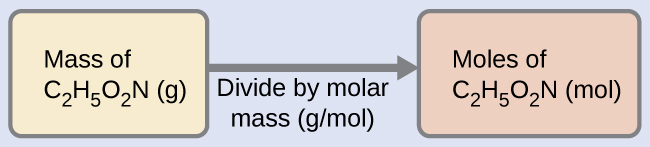The molar mass of glycine is required for this calculation, and it is computed in the same fashion as its molecular mass. One mole of glycine, C2H5O2N, contains 2 moles of carbon, 5 moles of hydrogen, 2 moles of oxygen, and 1 mole of nitrogen:

 2 C mass = 2 x 12.011 g/mol = 24.022 g/mol 5 H masses = 5 x 1.00794 g/mol = 5.0397 g/mol 2 O masses = 2 x 15.9994 g/mol = 31.9988 g/mol 1 N masses = 1 x 14.0067 g/mol = 14.0067 g/mol Total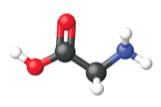= 75.067 g/mol = the molar mass of C2H5O2N

Figure 5. The average mass of a mole of glycine, C2H5O2N, is 75.067 g/mol, which is the sum of the average molar masses of each of its constituent atoms. The molecular structure of glycine.

The provided mass of glycine (~28 g) is a bit more than one-third the molar mass (~75 g/mol), so we would expect the computed result to be a bit greater than one-third of a mole (~0.33 mol). Dividing the compound’s mass by its molar mass yields:

$28.35 \;\rule[0.5ex]{0.5em}{0.1ex}\hspace{-0.5em}\text{g} \;\text{glycine} \; \times \frac{\text{mol glycine}}{75.067 \;\rule[0.25ex]{0.5em}{0.1ex}\hspace{-0.5em}\text{g}} = 0.3777 \;\text{mol of glycine}$

This result is consistent with our rough estimate.

Test Yourself
How many moles of sucrose, C12H22O11, are in a 25-g sample of sucrose?

0.073 mol

### Example 6

Vitamin C is a covalent compound with the molecular formula C6H8O6. The recommended daily dietary allowance of vitamin C for children aged 4–8 years is 1.42 × 10−4 mol. What is the mass of this allowance in grams?

Solution
As for elements, the mass of a compound can be derived from its molar amount as shown:The molar mass for this compound is computed to be 176.126 g/mol. The given number of moles is a very small fraction of a mole (~10−4 or one-ten thousandth); therefore, we would expect the corresponding mass to be about one-ten thousandth of the molar mass (~0.02 g). Performing the calculation, we get:

$1.42 \times 10^{-4} \;\rule[0.5ex]{1.75em}{0.1ex}\hspace{-1.75em}\text{mol} \;\text{vitamin C} \times \frac{176.126 \text{g}}{\rule[0.25ex]{1.25em}{0.1ex}\hspace{-1.25em}\text{mol} \;\text{vitamin C}} = 0.0250 \;\text{g of vitamin C}$

This is consistent with the anticipated result.

Test Yourself
What is the mass of 0.443 mol of hydrazine, N2H4?

14.2 g

Just as we can use the chemical formula to go between molecules of a compound and atoms of an element in the compound (and vice versa):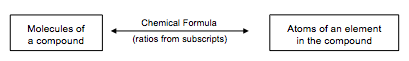we can also use chemical formulas as conversion factors to convert from moles of a compound to moles of an element in the compound (and vice versa).Be particularly careful when looking at the mass of an element in a compound. Remember, the only way you can change from molecule to atoms, or vice-versa, is by using the chemical formula, and the chemical formula relates ONLY to particles or moles, not to mass.

### Example 7

A packet of an artificial sweetener contains 40.0 mg of saccharin (C7H5NO3S), which has the structural formula: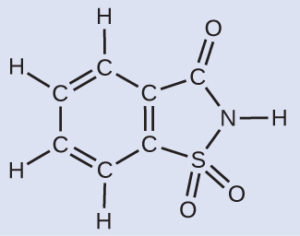Given that saccharin has a molar mass of 183.188 g/mol, how many saccharin molecules are in a 40.0-mg (0.0400-g) sample of saccharin? How many carbon atoms are in the same sample?

Solution
The number of molecules in a given mass of compound is computed by first deriving the number of moles, as demonstrated in Example 5, and then multiplying by Avogadro’s number: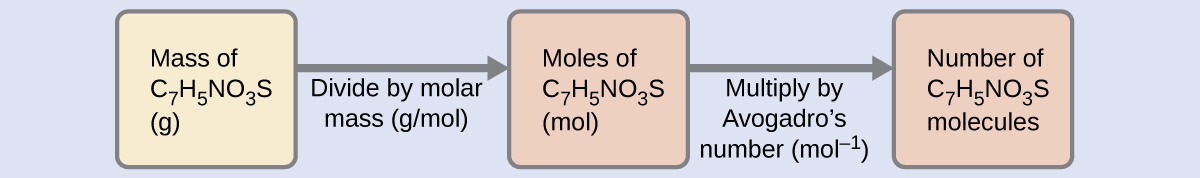Using the provided mass and molar mass for saccharin yields:

$0.0400 \;\rule[0.5ex]{0.5em}{0.1ex}\hspace{-0.5em}\text{g} \; \text{C}_7\text{H}_5\text{NO}_3\text{S} \times \frac{\rule[0.25ex]{1.25em}{0.1ex}\hspace{-1.25em}\text{mol} \;\text{C}_7\text{H}_5\text{NO}_3\text{S}}{183.188 \;\rule[0.25ex]{0.5em}{0.1ex}\hspace{-0.5em}\text{g} \;\text{C}_7\text{H}_5\text{NO}_3\text{S}} \times \frac{6.022 \times 10^{23} \;\text{C}_7\text{H}_5\text{NO}_3\text{S} \;\text{molecules}}{1\;\rule[0.25ex]{1.25em}{0.1ex}\hspace{-1.25em}\text{mol} \;\text{C}_7\text{H}_5\text{NO}_3\text{S}}$ $= \underline{1.31}49 \times 10^{20} \;\text{C}_7\text{H}_5\text{NO}_3\text{S} \;\text{molecules with 3 sig figs}$

The compound’s formula shows that each molecule contains seven carbon atoms, and so the number of C atoms in the provided sample is:

$\underline{1.31}49 \times 10^{20}\;\rule[0.5ex]{4.0em}{0.1ex}\hspace{-4.0em}\text{molecules}\; \text{C}_7\text{H}_5\text{NO}_3\text{S} \times \frac{7\;\text{C atoms}} {1 \;\rule[0.25ex]{3.0em}{0.1ex}\hspace{-3.0em}\text{molecules}\; \text{C}_7\text{H}_5\text{NO}_3\text{S}}$ $= \underline{9.20}43 \times 10^{21} \;\text{C molecules with 3 sig figs}$ $= 9.20 \times 10^{21} \;\text{C molecules}$

Test Yourself
How many C4H10 molecules are contained in 9.213 g of this compound? How many hydrogen atoms?

9.545 × 1022 molecules C4 H10; 9.545 × 1023 atoms H

### Counting Neurotransmitter Molecules in the Brain

The brain is the control center of the central nervous system (Figure 6). It sends and receives signals to and from muscles and other internal organs to monitor and control their functions; it processes stimuli detected by sensory organs to guide interactions with the external world; and it houses the complex physiological processes that give rise to our intellect and emotions. The broad field of neuroscience spans all aspects of the structure and function of the central nervous system, including research on the anatomy and physiology of the brain. Great progress has been made in brain research over the past few decades, and the BRAIN Initiative, a federal initiative announced in 2013, aims to accelerate and capitalize on these advances through the concerted efforts of various industrial, academic, and government agencies (more details available at www.whitehouse.gov/share/brain-initiative).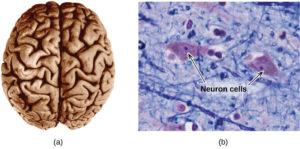Figure 6. (a) A typical human brain weighs about 1.5 kg and occupies a volume of roughly 1.1 L. (b) Information is transmitted in brain tissue and throughout the central nervous system by specialized cells called neurons (micrograph shows cells at 1600× magnification).

Specialized cells called neurons transmit information between different parts of the central nervous system by way of electrical and chemical signals. Chemical signaling occurs at the interface between different neurons when one of the cells releases molecules (called neurotransmitters) that diffuse across the small gap between the cells (called the synapse) and bind to the surface of the other cell. These neurotransmitter molecules are stored in small intracellular structures called vesicles that fuse to the cell wall and then break open to release their contents when the neuron is appropriately stimulated. This process is called exocytosis (see Figure 7). One neurotransmitter that has been very extensively studied is dopamine, C8H11NO2. Dopamine is involved in various neurological processes that impact a wide variety of human behaviors. Dysfunctions in the dopamine systems of the brain underlie serious neurological diseases such as Parkinson’s and schizophrenia.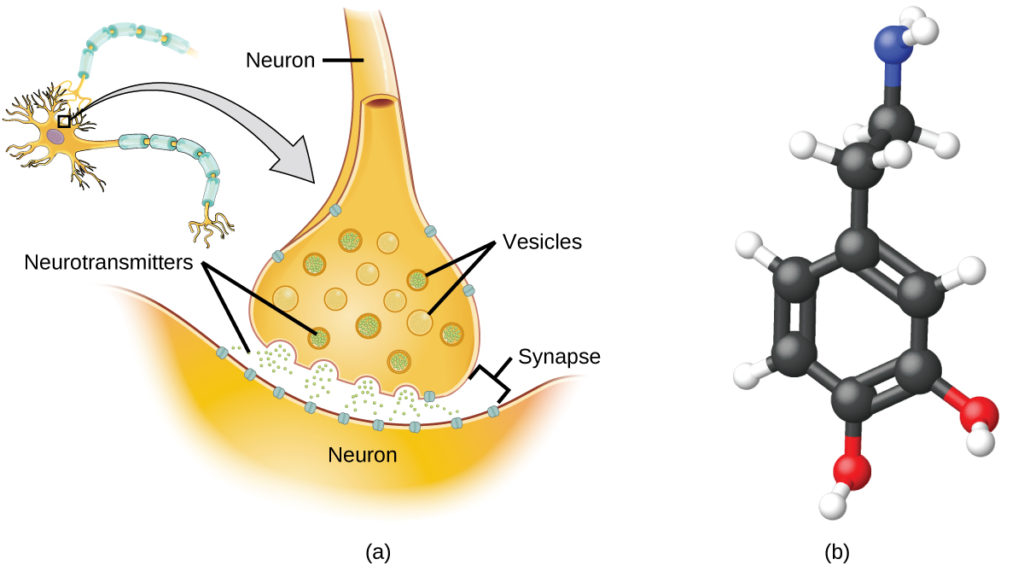Figure 7. (a) Chemical signals are transmitted from neurons to other cells by the release of neurotransmitter molecules into the small gaps (synapses) between the cells. (b) Dopamine, C8H11NO2, is a neurotransmitter involved in a number of neurological processes.

One important aspect of the complex processes related to dopamine signaling is the number of neurotransmitter molecules released during exocytosis. Since this number is a central factor in determining neurological response (and subsequent human thought and action), it is important to know how this number changes with certain controlled stimulations, such as the administration of drugs. It is also important to understand the mechanism responsible for any changes in the number of neurotransmitter molecules released—for example, some dysfunction in exocytosis, a change in the number of vesicles in the neuron, or a change in the number of neurotransmitter molecules in each vesicle.

Significant progress has been made recently in directly measuring the number of dopamine molecules stored in individual vesicles and the amount actually released when the vesicle undergoes exocytosis. Using miniaturized probes that can selectively detect dopamine molecules in very small amounts, scientists have determined that the vesicles of a certain type of mouse brain neuron contain an average of 30,000 dopamine molecules per vesicle (about 5 × 10−20 mol or 50 zmol). Analysis of these neurons from mice subjected to various drug therapies shows significant changes in the average number of dopamine molecules contained in individual vesicles, increasing or decreasing by up to three-fold, depending on the specific drug used. These studies also indicate that not all of the dopamine in a given vesicle is released during exocytosis, suggesting that it may be possible to regulate the fraction released using pharmaceutical therapies.

## Summary of using the mole idea: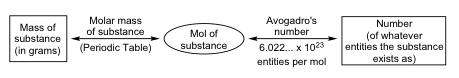Note:

• there is no direct conversion between number of atoms (or other entities) and grams of a substance. You need to first change to moles!
• which entities you’re expressing a number of will depend on what you are discussing. For example: Fe would be discussed in number of atoms, whereas Fe3+ would be expressed in ions, and H2O would be expressed in molecules.

### Example 8

a) How many atoms are present in a 25.0 g sample of Na?
b) What is the mass (in grams) of 5.00 x 1025atoms of Cr?

Solution

a) Convert grams to mol using molar mass, then to atoms with Avogadro’s no. (g$\longrightarrow$ mol$\longrightarrow$ atoms):

$25.0 \;\rule[0.5ex]{0.5em}{0.1ex}\hspace{-0.5em}\text{g Na}\; \times \frac{1\; \rule[0.25ex]{1.25em}{0.1ex}\hspace{-1.25em}\text{mol Na}} {22.9898 \;\rule[0.25ex]{0.5em}{0.1ex}\hspace{-0.5em}\text{g Na}} \times \frac{6.022 \times 10^{23}\; \text{atoms Na}}{1\;\rule[0.25ex]{1.25em}{0.1ex}\hspace{-1.25em}\text{mol of Na}}$ $= 6.55 \times 10^{23}\; \text{atoms of sodium}$

b) Now we are going from atoms $\longrightarrow$mol $\longrightarrow$ g

$5.00\times 10^{25}\; \rule[0.5ex]{2.0em}{0.1ex}\hspace{-2.0em}\text{atoms of Cr}\; \times \frac{1\; \rule[0.25ex]{1.25em}{0.1ex}\hspace{-1.25em}\text{mol Cr}} {6.022 \times 10^{23} \;\rule[0.25ex]{3.0em}{0.1ex}\hspace{-3.0em}\text{atoms Cr}} \times \frac{51.996\; \text{g Cr}}{1\;\rule[0.25ex]{1.25em}{0.1ex}\hspace{-1.25em}\text{mol of Cr}}$ $= 4.32 \times 10^{3}\; \text{g of chromium}$

Test Yourself

How many atoms are present in 21.2 mg of Ag?

1.18×1020 atoms of Ag

Notice that the Test Yourself  question above included a metric conversion. We can add metric and other conversions from Chapter 2 to our “road maps”.  Remember that there is often more than one approach for a question.

#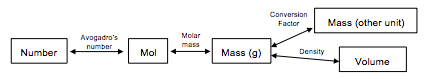### Example 9

How many atoms are in a piece of gold measuring 1 cm x 2 cm x 0.5 cm?
(Density of gold is 19.32 g/mL)

Solution

Our “road map” for this question:

Measurements $\longrightarrow$ volume $\longrightarrow$ g $\longrightarrow$ mol $\longrightarrow$ atoms

Keep in mind that 1 cm= 1 mL, we use the density to get the mass based on the measurements, and then follow the standard procedure to atoms using the molar mass and Avogadro’s number.

1 cm x 2 cm x 0.5 cm = 1 cm3 of Au

$1\; \rule[0.75ex]{1.25em}{0.1ex} \hspace{-1.25em} \text{cm}^3\; \times \frac{1\; \rule[0.5ex]{1.0em}{0.1ex} \hspace{-1.0em} \text{mL Au}} {1\; \rule[0.5ex]{1.25em}{0.1ex} \hspace{-1.25em} \text{cm}^3 \text{ Au}} \times \frac{19.32\; \rule[0.5ex]{0.5em}{0.1ex} \hspace{-0.5em}\text{g Au}} {1\; \rule[0.5ex]{0.8em}{0.1ex} \hspace{-0.8em} \text{mL Au}} \times \frac{1\; \rule[0.5ex]{1.25em}{0.1ex} \hspace{-1.25em} \text{mol Au}} {196.967\; \rule[0.5ex]{0.5em}{0.1ex} \hspace{-0.5em} \text{g Au}} \times \frac{6.022 \times 10^{23}\; \text{atoms of Au}}{1\; \rule[0.5ex]{1.25em}{0.1ex} \hspace{-1.25em} \text{mol Au}} = 6 \times 10^{22}\; \text{atoms of Au}$

Test Yourself

The density of mercury (Hg) is 13.53 g/mL. How many litres will 4.2 x 1021 atoms of Hg occupy?

1.0×10-4 L

## More Worked Out Problems

### Example 10

How many molecules are present in 2.76 mol of H2O? How many atoms is this?

Solution

The definition of a mole is an equality that can be used to construct a conversion factor. Also, because we know that there are three atoms in each molecule of H2O, we can also determine the number of atoms in the sample.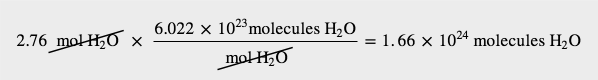To determine the total number of atoms, we have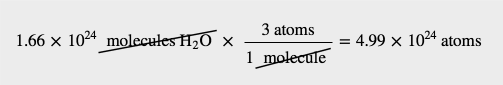Test Yourself

How many molecules are present in 4.61 × 10−2 mol of O2?

2.78 × 1022 molecules

### Example 11

What is the molar mass of C6H12O6?

Solution

To determine the molar mass, we simply add the atomic masses of the atoms in the molecular formula but express the total in grams per mole, not atomic mass units. The masses of the atoms can be taken from the periodic table:

 6 C = 6 × 12.011 = 72.066 12 H = 12 × 1.00794 = 12.09528 6 O = 6 × 15.9994 = 95.9964 TOTAL = 180.158 g/mol

Per convention, the unit grams per mole is written as a fraction.

Test Yourself

What is the molar mass of AgNO3?

169.873 g/mol

### Example 12

What is the mass of 3.56 mol of HgCl2? The molar mass of HgCl2 is 271.50 g/mol.

Solution

Use the molar mass as a conversion factor between moles and grams. Because we want to cancel the mole unit and introduce the gram unit, we can use the molar mass as given:

$3.56\; \rule[0.5ex]{1.25em}{0.1ex}\hspace{-1.25em}\text{mol}\; \text{HgCl}_2 \times \frac{271.50\; \text{g HgCl}_2} {1 \;\rule[0.25ex]{1.25em}{0.1ex}\hspace{-1.25em}\text{mol}\; \text{HgCl}_2}$ $= 967\; \text{g}\; \text{HgCl}_2$

Test Yourself

What is the mass of 33.7 mol of H2O?

607 g

### Example 13

How many moles of H2O are present in 240.0 g of water (about the mass of a cup of water)?

Solution

Use the molar mass of H2O as a conversion factor from mass to moles. The molar mass of water is (1.00794 + 1.00794 + 15.9994) = 18.0153 g/mol. However, because we want to cancel the gram unit and introduce moles, we need to take the reciprocal of this quantity, or 1 mol/18.0153 g:

$240.0\; \rule[0.5ex]{0.5em}{0.1ex}\hspace{-0.5em}\text{g}\; \text{H}_2\text{O} \times \frac{1\; \text{mol H}_2\text{O}} {18.0153\; \rule[0.25ex]{0.5em}{0.1ex}\hspace{-0.5em}\text{g}\; \text{H}_2\text{O}}$ $= 13.32\; \text{mol}\; \text{H}_2\text{O}$

Test Yourself

How many moles are present in 35.6 g of H2SO4 (molar mass = 98.079 g/mol)?

0.363 mol

### Example 14

The density of ethanol is 0.789 g/mL. How many moles are in 100.0 mL of ethanol? The molar mass of ethanol is 46.069 g/mol.

Solution

Here, we use density to convert from volume to mass and then use the molar mass to determine the number of moles.

$100.0\; \rule[0.5ex]{0.8em}{0.1ex}\hspace{-0.8em}\text{mL ethanol}\; \times \frac{0.789\; \text{g}} {1\; \rule[0.25ex]{0.8em}{0.1ex}\hspace{-0.8em}\text{mL}} \times \frac{1\; \text{mol}} {46.069\; \rule[0.25ex]{0.5em}{0.1ex}\hspace{-0.5em}\text{g}}$ $= 1.71\; \text{mol ethanol}$

Test Yourself

If the density of benzene, C6H6, is 0.879 g/mL, how many moles are present in 17.9 mL of benzene?

0.201 mol

## Key Concepts and Summary

A convenient amount unit for expressing very large numbers of atoms or molecules is the mole. Experimental measurements have determined the number of entities composing 1 mole of substance to be 6.022 × 1023, a quantity called Avogadro’s number. The mass in grams of 1 mole of substance is its molar mass. Due to the use of the same reference substance in defining the atomic mass unit and the mole, the formula mass (amu) and molar mass (g/mol) for any substance are numerically equivalent (for example, one H2O molecule weighs approximately 18 amu and 1 mole of H2O molecules weighs approximately 18 g).

### Exercises

1. Write a sentence that describes how to determine the number of moles of a compound in a known mass of the compound if we know its molecular formula.

2. Which contains the greatest mass of oxygen: 0.75 mol of ethanol (C2H5OH), 0.60 mol of formic acid (HCO2H), or 1.0 mol of water (H2O)? Explain why.

3. How are the molecular mass and the molar mass of a compound similar and how are they different?

4. Calculate the molar mass of each of the following:

a) S8      b) C5H12      c) Sc2(SO4)3

d) CH3COCH3 (acetone)      e) C6H12O6 (glucose)

5. Calculate the molar mass of each of the following:

a) the anesthetic halothane, C2HBrClF3

b) the herbicide paraquat, C12H14N2Cl2

c) caffeine, C8H10N4O2

d) urea, CO(NH2)2

e) a typical soap, C17H35CO2Na

6. Determine the mass of each of the following:

a) 0.0146 mol KOH

b) 10.2 mol ethane, C2H6

c) 1.6 × 10−3 mol Na2 SO4

d) 6.854 × 103 mol glucose, C6 H12 O6

e) 2.86 mol Co(NH3)6Cl3

7. Determine the mass of each of the following:

a) 2.345 mol LiCl

b) 0.0872 mol acetylene, C2H2

c) 3.3 × 10−2 mol Na2 CO3

d) 1.23 × 103 mol fructose, C6 H12 O6

e) 0.5758 mol FeSO4•(H2O)7

8. Determine the mass in grams of each of the following:

a) 0.600 mol of oxygen atoms

b) 0.600 mol of oxygen molecules, O2

c) 0.600 mol of ozone molecules, O3

9. Determine the number of atoms and the mass of zirconium, silicon, and oxygen found in 0.3384 mol of zircon, ZrSiO4, a semiprecious stone.

10. Determine which of the following contains the greatest mass of aluminum: 122 g of AlPO4, 266 g of Al2C16, or 225 g of Al2S3.

11. The Cullinan diamond was the largest natural diamond ever found (January 25, 1905). It weighed 3104 carats (1 carat = 200 mg). How many carbon atoms were present in the stone?

12. A certain nut crunch cereal contains 11.0 grams of sugar (sucrose, C12H22O11) per serving size of 60.0 grams. How many servings of this cereal must be eaten to consume 0.0278 moles of sugar?

13. Which of the following represents the least number of molecules?

a) 20.0 g of H2O (18.02 g/mol)

b) 77.0 g of CH4 (16.06 g/mol)

c) 68.0 g of CaH2 (42.09 g/mol)

d) 100.0 g of N2O (44.02 g/mol)

e) 84.0 g of HF (20.01 g/mol)

14. How many atoms are present in 4.55 mol of Fe?

15. How many molecules are present in 2.509 mol of H2S?

16. How many moles are present in 3.55 × 1024 Pb atoms?

17. How many moles are present in 1.00 × 1023 PF3 molecules?

18. Determine the molar mass of each substance.

a)  Si      b)  SiH4      c)  K2O

19.  Determine the molar mass of each substance.

a)  Al      b)  Al2O3      c)  CoCl3

20.  What is the mass of 4.44 mol of Rb?

21.  What is the mass of 12.34 mol of Al2(SO4)3?

22.  How many moles are present in 45.6 g of CO?

23.  How many moles are present in 1.223 g of SF6?

24.  How many moles are present in 54.8 mL of mercury if the density of mercury is 13.6 g/mL?

1. Use the molecular formula to find the molar mass; to obtain the number of moles, divide the mass of compound by the molar mass of the compound expressed in grams.

2. Formic acid. Its formula has twice as many oxygen atoms as the other two compounds (one each). Therefore, 0.60 mol of formic acid would be equivalent to 1.20 mol of a compound containing a single oxygen atom.

3. The two masses have the same numerical value, but the units are different: The molecular mass is the mass of 1 molecule while the molar mass is the mass of 6.022 × 1023 molecules.

4. a) 256.528 g/mol         b) 72.150 g mol−1         c) 378.103 g mol−1

d) 58.080 g mol−1          e) 180.158 g mol−1

5. a) 197.382 g mol−1         b) 257.162 g mol−1         c) 194.193 g mol−1

d) 60.056 g mol−1         e) 306.464 g mol−1

6. a) 0.819 g         b) 307 g         c) 0.23 g         d) 1.235 × 106 g (1235 kg)

e) 765 g

7. a) 99.41         b) 2.27 g         c) 3.5 g         d) 222 kg         e) 160.1 g

8. a) 9.60 g         b) 19.2 g         c) 28.8 g

9. zirconium: 2.038 × 1023 atoms; 30.87 g; silicon: 2.038 × 1023 atoms; 9.504 g; oxygen: 8.151 × 1023 atoms; 21.66 g

10. 122 g of AlPO4 = 27.0 g of Al        266 g of Al2Cl= 53.8 g of Al         225 g of Al2S= 80.8 g Al; therefore 225 g of Al2Shas the greatest mass of Al.

11. 3.113 × 1025 C atoms

12. 0.865 servings, or about 1 serving.

13. 20.0 g H2O represents the least number of molecules since it has the least number of moles.

14. 2.74 × 1024 atoms

15. 1.511 × 1024 molecules

16. 5.90 mol

17. 0.166 mol

18. a)  28.0855 g          b)  32.1172 g            c)  94.1960 g

19. a)  26.9815 g          b)  101.9612 g          c)  165.2913 g

20. 379 g

21. 4,222 g

22. 1.63 mol

23. 0.008373 mol

24. 3.72 mol

## Glossary

Avogadro’s number (NA): experimentally determined value of the number of entities comprising 1 mole of substance, equal to 6.022 × 1023 mol−1

formula mass: sum of the average masses for all atoms represented in a chemical formula; for covalent compounds, this is also the molecular mass

molar mass: mass in grams of 1 mole of a substance

mole: amount of substance containing the same number of atoms, molecules, ions, or other entities as the number of atoms in exactly 12 grams of 12C

1. Omiatek, Donna M., Amanda J. Bressler, Ann-Sofie Cans, Anne M. Andrews, Michael L. Heien, and Andrew G. Ewing. “The Real Catecholamine Content of Secretory Vesicles in the CNS Revealed by Electrochemical Cytometry.” Scientific Report 3 (2013): 1447, accessed January 14, 2015, doi:10.1038/srep01447.